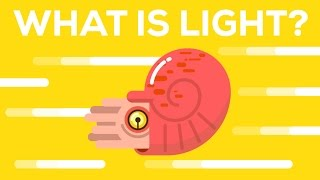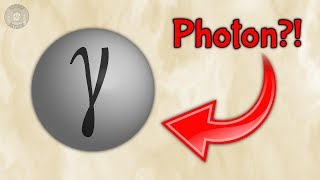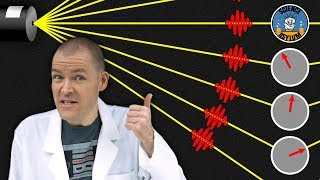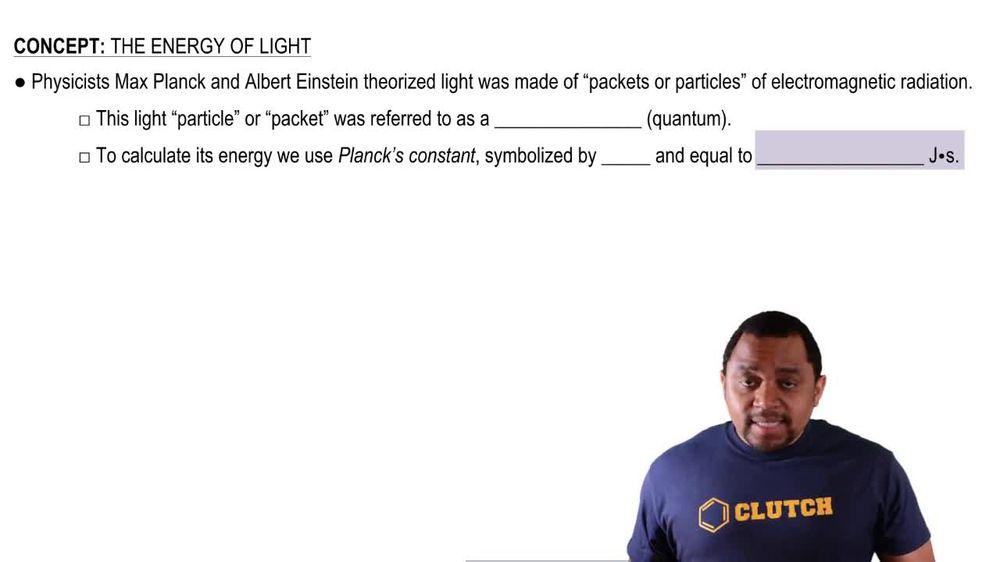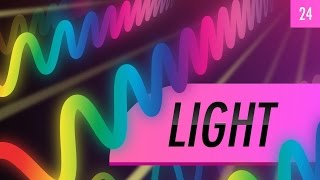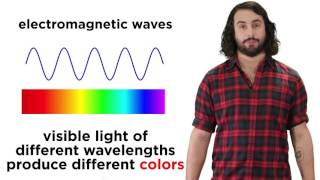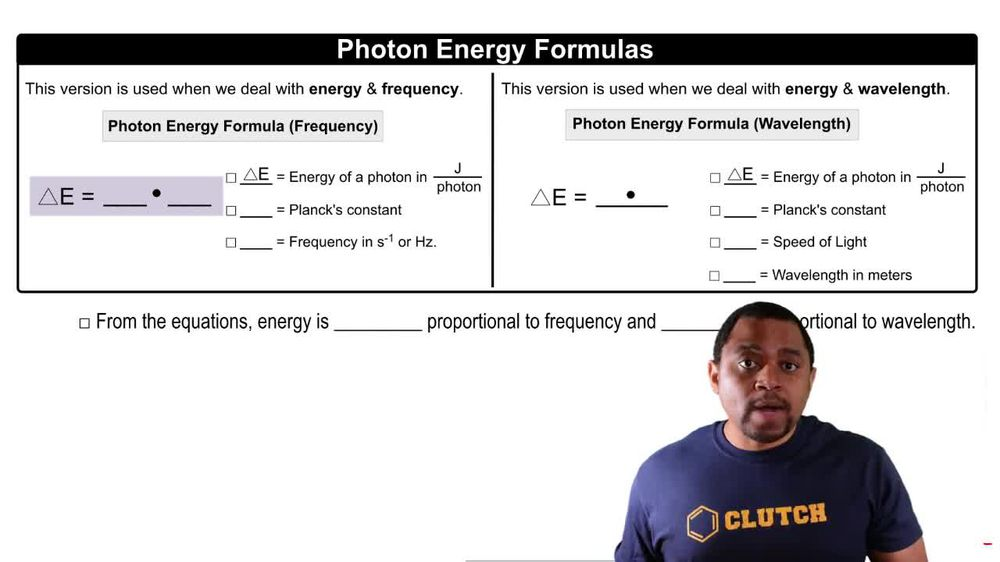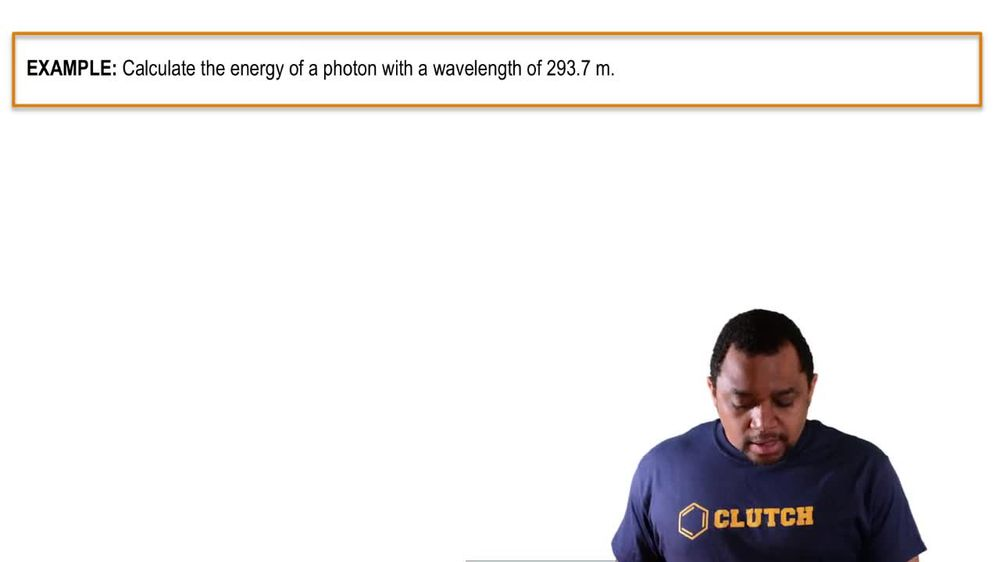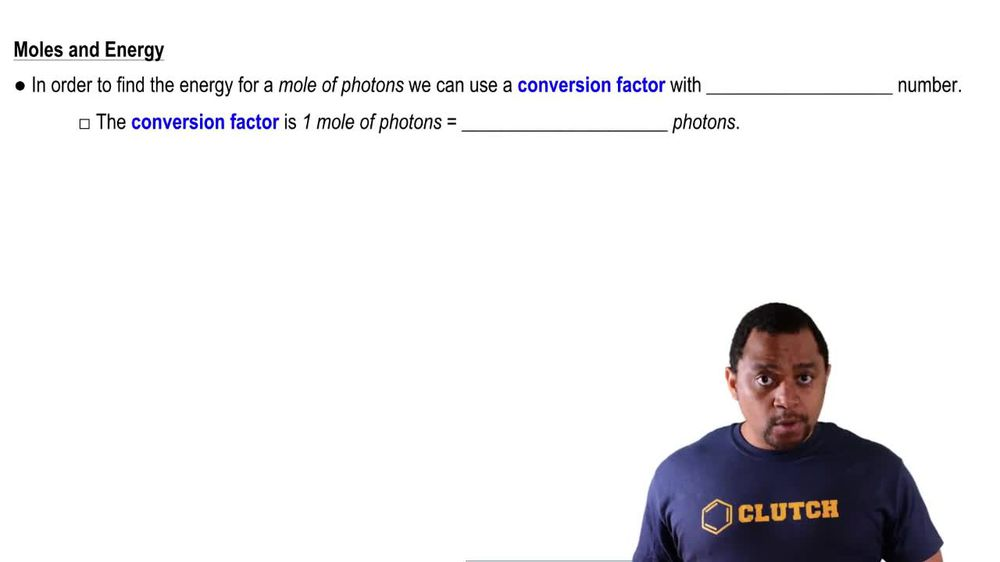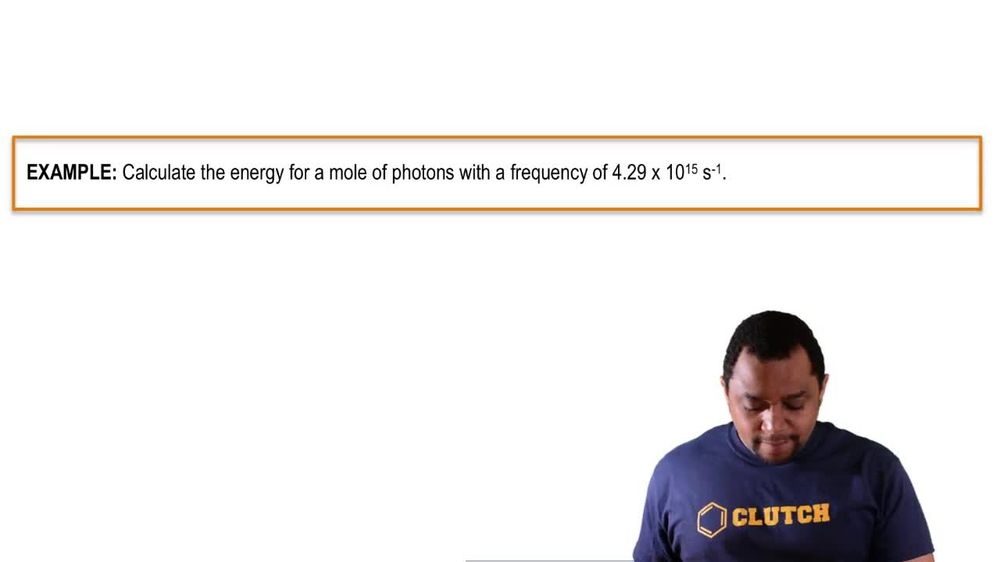Start typing, then use the up and down arrows to select an option from the list.
1. 9. Quantum Mechanics2. The Energy of Light# The Energy of Light

by Jules Bruno
186 views
0
Now, up to this point, we know how to calculate the energy off. One photon on. We know unity would be jewels for photon. Now, in order to find the energy for Mullah photons, we can use the conversion factor with avocados number. Now here, the conversion factor would be one mullah Photons equals 6.22 times 10 to the 23 photons. So we know that that value represents avocados number. So just remember, when we calculate the jewels per photon from our formulas, we can use avocados number to convert it into jewels per mole of photons. Now we know this connection. Move onto the next video and let's put it to work.